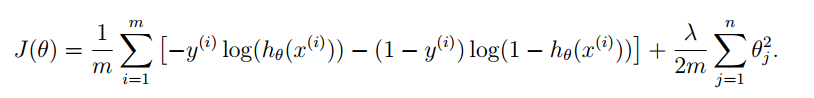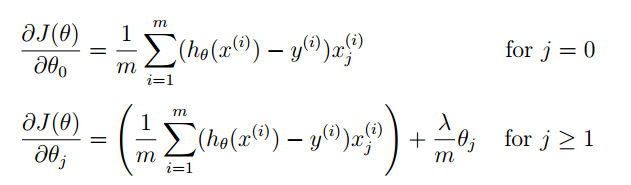# 机器学习：过拟合与欠拟合问题

(在苹果系统下，如果文章中的图片不能正常显示，请升级Safari浏览器到最新版本，或者使用Chrome、Firefox浏览器打开。)function [J, grad] = CostFunction(theta, X, y)
m = length(y); % 训练样本数
J = 0;

theta_1=[0;theta(2:end)];    % 先把theta(1)拿掉，不参与正则化
lambda = 1;    % 正则因子
J = sum(-y .* log(sigmoid(X * theta)) - (1 - y) .* log(1 - sigmoid(X * theta)) )/m  + lambda/(2*m) * theta_1' * theta_1;
grad = (X' * (sigmoid(X * theta) - y))/m + lambda/m * theta_1;

end


 版权声明本博客的文章除特别说明外均为原创，本人版权所有。欢迎转载，转载请注明作者及来源链接，谢谢。本文地址: https://blog.ailemon.net/2017/02/22/overfitting-and-underfitting/ All articles are under Attribution-NonCommercial-ShareAlike 4.0## “机器学习：过拟合与欠拟合问题”上的1条回复Tingting Li说：